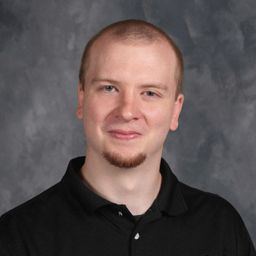Join our free STEM summer bootcamps taught by experts. Space is limited.Register Here 🏕# Derrick H.

University of Central Missouri

## Biography

I am a full-time High School Mathematics Teacher in Missouri. I am most familiar with teaching Algebra (any level) and Pre-calculus.

## Education

BA Secondary Mathematics Education
University of Central Missouri

## Topics Covered

Trigonometry
Introduction to Vectors
Complex Numbers
Sampling and Data
The Normal Distribution
Introduction to Combinatorics and Probability
Functions
Exponential and Logarithmic Functions
Linear Regression and Correlation
Fractions and Mixed Numbers
Decimals
Equations and Inequalities
Probability
Probability Topics
Polynomials
Rational Functions
Introduction to Algebra
Logic
Volume
Systems of Equations and Inequalities
Graphs and Statistics
The Language of Algebra
Exponents and Polynomials
Functions
Introduction to Matrices
Introduction to Sequences and Series
Introduction to Combinatorics and Probability
Linear Functions